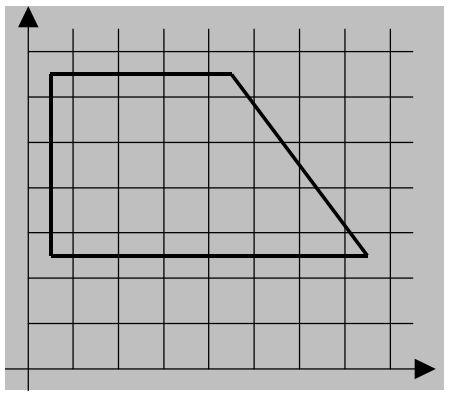시간 제한메모리 제한제출정답맞힌 사람정답 비율
1 초 128 MB111100.000%

## 문제

Write a program to find a convex polygon whose sides have the given lengths.

In this task, we consider a polygon to be convex if all its inner angles are strictly greater than 0 degrees and strictly less than 180 degrees.

## 입력

The first line of the file contains an integer N, the number of vertices of the polygon (3 ≤ N ≤ 1000). Each of the following N lines contains an integer ai, the length of one side of the polygon (1 ≤ ai ≤ 10,000).

## 출력

If the desired polygon can be constructed, the output should contain exactly N lines. Each line should contain two real numbers xi and yi (|xi| ≤ 10,000,000, |yi| ≤ 10,000,000) such that by connecting the points (xi, yi) and (xi+1, yi+1) for all 1 ≤ i < N and additionally the points (xN, yN) and (x1, y1) with line segments, we obtain a convex polygon. The lengths of the line segments must be equal to the numbers given in the input, but not necessarily in the same order.

The vertices of the constructed polygon can be listed either clockwise or counterclockwise.

If the polygon cannot be constructed, print NO SOLUTION on the single line of the output.

The grading program considers two lengths equal if they differ by less than 0.001. Any standard floating point format is acceptable.

## 예제 입력 1

4
7
4
5
4


## 예제 출력 1

0.5 2.5
7.5 2.5
4.5 6.5
0.5 6.5


## 힌트